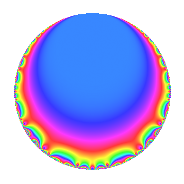# Properties

 Label 95.1.d.aLevel 95 Weight 1 Character orbit 95.d Self dual Yes Analytic conductor 0.047 Analytic rank 0 Dimension 1 Projective image $$D_{2}$$ CM/RM disc. -19, -95, 5 Inner twists 4

# Related objects

## Newspace parameters

 Level: $$N$$ = $$95 = 5 \cdot 19$$ Weight: $$k$$ = $$1$$ Character orbit: $$[\chi]$$ = 95.d (of order $$2$$ and degree $$1$$)

## Newform invariants

 Self dual: Yes Analytic conductor: $$0.0474111762001$$ Analytic rank: $$0$$ Dimension: $$1$$ Coefficient field: $$\mathbb{Q}$$ Coefficient ring: $$\mathbb{Z}$$ Coefficient ring index: $$1$$ Projective image $$D_{2}$$ Projective field Galois closure of $$\Q(\sqrt{5}, \sqrt{-19})$$ Artin image size $$8$$ Artin image $D_4$ Artin field Galois closure of 4.2.475.1

## $q$-expansion

 $$f(q)$$ $$=$$ $$q$$ $$\mathstrut -\mathstrut q^{4}$$ $$\mathstrut +\mathstrut q^{5}$$ $$\mathstrut -\mathstrut q^{9}$$ $$\mathstrut +\mathstrut O(q^{10})$$ $$q$$ $$\mathstrut -\mathstrut q^{4}$$ $$\mathstrut +\mathstrut q^{5}$$ $$\mathstrut -\mathstrut q^{9}$$ $$\mathstrut -\mathstrut 2q^{11}$$ $$\mathstrut +\mathstrut q^{16}$$ $$\mathstrut +\mathstrut q^{19}$$ $$\mathstrut -\mathstrut q^{20}$$ $$\mathstrut +\mathstrut q^{25}$$ $$\mathstrut +\mathstrut q^{36}$$ $$\mathstrut +\mathstrut 2q^{44}$$ $$\mathstrut -\mathstrut q^{45}$$ $$\mathstrut +\mathstrut q^{49}$$ $$\mathstrut -\mathstrut 2q^{55}$$ $$\mathstrut -\mathstrut 2q^{61}$$ $$\mathstrut -\mathstrut q^{64}$$ $$\mathstrut -\mathstrut q^{76}$$ $$\mathstrut +\mathstrut q^{80}$$ $$\mathstrut +\mathstrut q^{81}$$ $$\mathstrut +\mathstrut q^{95}$$ $$\mathstrut +\mathstrut 2q^{99}$$ $$\mathstrut +\mathstrut O(q^{100})$$

## Character Values

We give the values of $$\chi$$ on generators for $$\left(\mathbb{Z}/95\mathbb{Z}\right)^\times$$.

 $$n$$ $$21$$ $$77$$ $$\chi(n)$$ $$-1$$ $$-1$$

## Embeddings

For each embedding $$\iota_m$$ of the coefficient field, the values $$\iota_m(a_n)$$ are shown below.

For more information on an embedded modular form you can click on its label.

Label $$\iota_m(\nu)$$ $$a_{2}$$ $$a_{3}$$ $$a_{4}$$ $$a_{5}$$ $$a_{6}$$ $$a_{7}$$ $$a_{8}$$ $$a_{9}$$ $$a_{10}$$
94.1
 0
0 0 −1.00000 1.00000 0 0 0 −1.00000 0
 $$n$$: e.g. 2-40 or 990-1000 Significant digits: Format: Complex embeddings Normalized embeddings Satake parameters Satake angles

## Inner twists

Char. orbit Parity Mult. Self Twist Proved
1.a Even 1 trivial yes
5.b Even 1 RM by $$\Q(\sqrt{5})$$ yes
19.b Odd 1 CM by $$\Q(\sqrt{-19})$$ yes
95.d Odd 1 CM by $$\Q(\sqrt{-95})$$ yes

## Hecke kernels

This newform can be constructed as the kernel of the linear operator $$T_{2}$$ acting on $$S_{1}^{\mathrm{new}}(95, [\chi])$$.